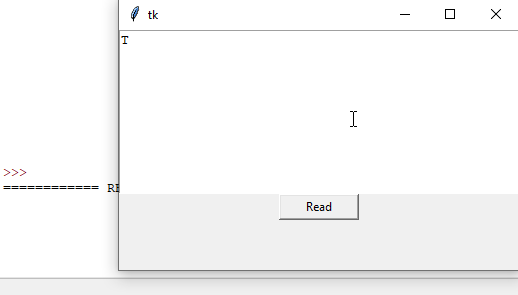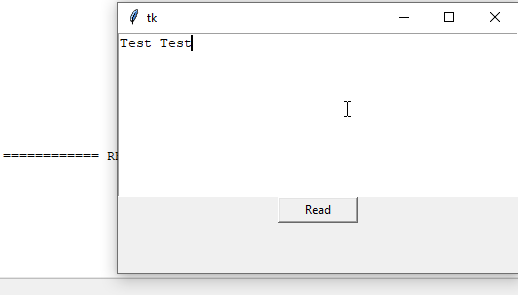# Get the Input From Tkinter Text Box

Tkinter Text widget has `get()` method to return the input from the text box, which has `start` position argument, and an optional `end` argument to specify the end position of the text to be fetched.

``````get(start, end=None)
``````

If `end` is not given, only one character specified at the `start` position will be returned.

## Example Code to Get the Input From Tkinter Text Widget

``````import tkinter as tk
root = tk.Tk()
root.geometry("400x240")

def getTextInput():
result=textExample.get("1.0","end")
print(result)

textExample=tk.Text(root, height=10)
textExample.pack()
command=getTextInput)

root.mainloop()
``````
``````result=textExample.get("1.0", "end")
``````

The position of the first character in the `Text` widget is `1.0`, and could be referred to as a number `1.0` or a string `"1.0"`.

`"end"` means that it reads the input until the end of the `Text` box. We could also use `tk.END` instead of the string `"end"` here.The tiny issue if we specify `"end"` as the end position of the text to be returned, it includes also the newline `\n` character at the end of the text string, as you could see from the above animation.

We could change the `"end"` argument of the `get` method to be the `"end-1c"` if we don’t want the newline in the returned input.

`"end-1c"` means the position is one character ahead of `"end"`.

## Example Code to Fetch the Input Without `newline` at the End From Tkinter Text Widget

``````import tkinter as tk
root = tk.Tk()
root.geometry("400x240")

def getTextInput():
result=textExample.get(1.0, tk.END+"-1c")
print(result)

textExample=tk.Text(root, height=10)
textExample.pack()
command=getTextInput)

root.mainloop()
``````Here, we could also use `tk.END+"-1c"` besides `"end-1c"` to eliminate the last character - `\n`, because `tk.END = "end"`, therefore `tk.END+"-1c"` is equal to `"end"+"-1c"="end-1c"`.

Contribute
DelftStack is a collective effort contributed by software geeks like you. If you like the article and would like to contribute to DelftStack by writing paid articles, you can check the write for us page.

## Related Article - Tkinter Text

• Delete Tkinter Text Box's Contents